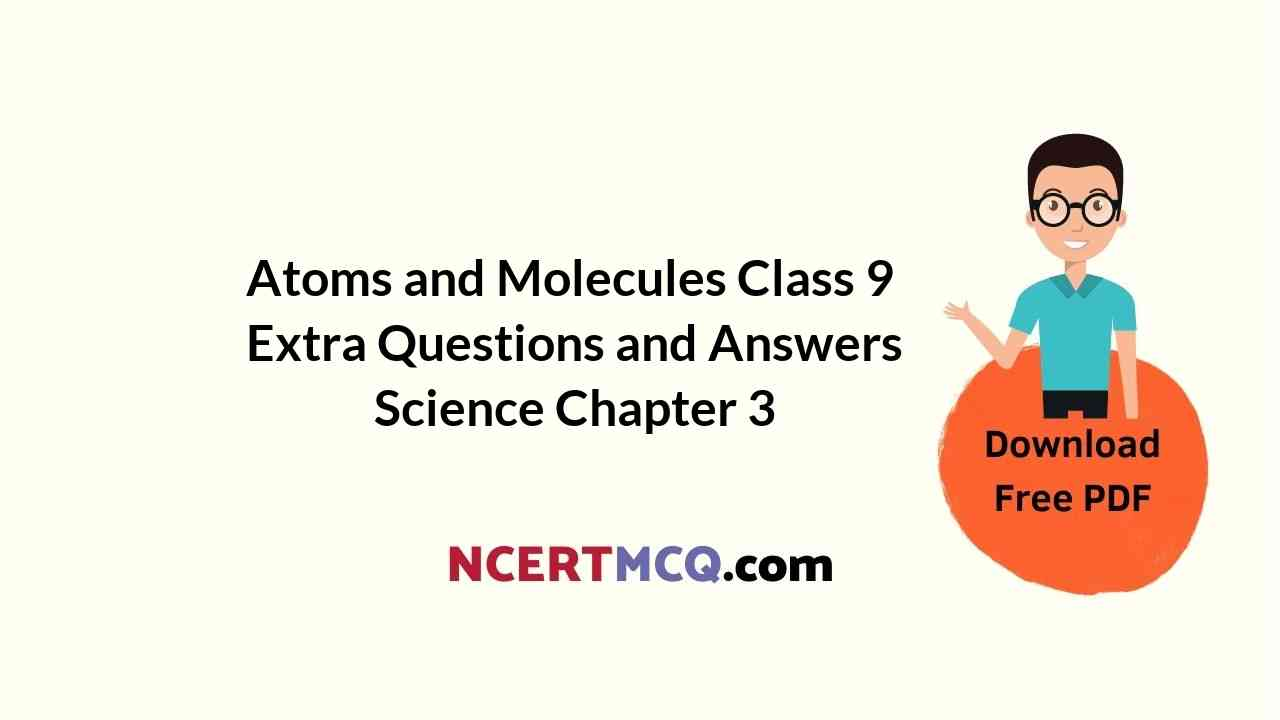In this page, we are providing Online Education for Atoms and Molecules Class 9 Extra Questions and Answers Science Chapter 3 pdf download. NCERT Extra Questions for Class 9 Science Chapter 3 Atoms and Molecules with Answers will help to score more marks in your CBSE Board Exams. https://ncertmcq.com/extra-questions-for-class-9-science/

## Online Education for Class 9 Science Chapter 3 Extra Questions and Answers Atoms and Molecules

Extra Questions for Class 9 Science Chapter 3 Atoms and Molecules with Answers Solutions

### Atoms and Molecules Class 9 Extra Questions Very Short Answer Type

Atoms And Molecules Class 9 Extra Questions Question 1.
Define atomic mass unit.
Atomic mass unit of an element is one twelfth (1/12th) of the mass of one atom of carbon-12.

Class 9 Science Chapter 3 Extra Questions And Answers Question 2.
Write the valency of sulphur in H2S, SO2 and SO3.
The valency of sulphur in H2S, SO2 and SO3 are 2, 4, and 6 respectively.

Atoms And Molecules Extra Questions Question 3.
What is the difference between 2H and H2?
2H means two atoms of hydrogen, H2 means a molecule of hydrogen which contains two atoms.

Class 9 Atoms And Molecules Extra Questions Question 4.
Where do we use the words mole and mol?
We use the word mole to define the number of atoms, molecules, ions or particles having a mass equal to its atomic or molecular mass in grams, while as a unit, we call it mol.

Extra Questions On Atoms And Molecules Class 9 Question 5.
The valency of an element A is 4. Write the formula of its oxide.
The formula of its oxide is A2O2 or AO2.

Extra Questions Of Atoms And Molecules Class 9 Question 6.
Why are Dalton’s symbols not used in chemistry?
Dalton was the first scientist to use the symbol for the name of the elements in a specific sense but it was difficult to memorise and in use, so Dalton’s symbols are not used in chemistry.

Class 9 Science Ch 3 Extra Questions Question 7.
Which postulate of Dalton’s Atomic theory is the basis of law of conservation of mass?
“Atoms can neither be created nor destroyed a physical or a chemical change”.

Class 9 Chapter 3 Science Extra Questions Question 8.
Calculate the formula unit mass of CaCl2. [NCERT Exemplar]
Atomic mass of Ca + (2 × atomic mass of Cl)
= 40 + 2 × 35.5 = 40 + 71 = 111 u.

Ch 3 Science Class 9 Extra Questions Question 9.
What does the symbol ‘u’ represent?
The symbol ‘u’ represents unified mass.

Class 9 Chemistry Chapter 3 Extra Questions Question 10.
Avogadro’s number represents how many particles?
Avogadro’s numbers (N0) represents 6.022 × 1023 particles.

Mole Concept Class 9 Extra Questions Question 11.
Formula of the carbonate of a metal M is M2CO3. Write the formula of its chloride.
The valency of the metal (M) in M2CO3 is (1+) i.e., metal exists as M+ ion. Therefore, the formula of metal chloride is MCl.

Atom And Molecules Class 9 Extra Questions Question 12.
Sample A contains one gram molecule of oxygen molecules and Sample B contains one mole of oxygen molecules. What is the ratio of the number of molecules in both the samples?
One gram molecules is the same as one gram mole of a substance. Therefore, both the samples A and B contain the same number of molecules (6.022 × 10)23 and the ratio is 1: 1.

Class 9 Ch 3 Science Extra Questions Question 13.
Name the compound Al2(SO4)3 and mention the ions present in it.
The compound is called aluminium sulphate; cation: Al3+; anion: (SO4)2-

Class 9 Science Chapter 3 Extra Questions Question 14.
Chemical symbol of sodium is Na. What is its Latin name?
Natrium.

Class 9 Science Atoms And Molecules Extra Questions Question 15.
Give one example of a polyatomic cation.
NH4+ (Ammonium ion).

Atoms And Molecules Class 9 Extra Numerical Questions Question 16.
MNO3 is the formula of nitrate of metal M. Write the formula of its oxide.
M2O

Ncert Class 9 Science Chapter 3 Extra Questions Question 17.
A vessel contains W molecules of oxygen at a certain temperature and pressure. How many molecules of sulphur dioxide can the vessel accommodate at the same temperature and pressure?
N molecules.

Atoms And Molecules Class 9 Extra Questions With Answers Question 18.
What do you understand by a polyatomic ion? Give two examples.
A group of atoms carrying positive or negative charge is called a polyatomic ion, e.g., NO3, SO42-, NH4+

Chapter 3 Science Class 9 Extra Questions Question 19.
Give two examples each of bivalent cations and bivalent anions.
Bivalent cations = Zn2+, Ca2+. Bivalent anions = SO42-, C32-.

Science Class 9 Chapter 3 Extra Questions Question 20.
What is ‘molar volume’? What is its value?
The volume occupied by one mole of a gas under standard conditions of temperature and pressure, i.e., STP conditions (0°C and 1 atmosphere i.e., pressure) is called molar volume of the gas. Its value is 22.4 L at STP.

Question 21.
What is the valency of calcium in CaCO3?
The valency of Ca in CaCO3 is 2+ [i.e. Ca2+].

Question 22.
Can we regard sodium as a monoatomic element?
No, sodium is a metal and does not exist as a single atom.

Question 23.
What happens to an element ‘A’ if its atom gains two electrons?
It changes to a divalent anion [A2-].

Question 24.
Do atoms in some of the elements have actually fractional mass?
No, the atoms do not have fractional mass. Only their average comes out to be in fraction in elements which exists as isotopes.

### Atoms and Molecules Class 9 Extra Questions Short Answer Type 1

Question 1.
Cinnabar (HgS) is a prominent ore of mercury. How many grams of mercury are present in 225 g of pure HgS? Molar mass of Hg and S are 200.6 g mol-1 and 32 g mol-1 respectively. [NCERT Exemplar]
Molar mass of HgS = 200.6 + 32 = 232.6 g mol-1
Mass of Hg in 232.6 g of HgS = 200.6 g
Mass of Hg in 225 g of HgS = $$\frac { 200.6 }{ 232.6 }$$ × 225 = 194.05 g.

Question 2.
A gold sample contains 90% of gold and the rest copper. How many atoms of gold are present in one gram of this sample of gold? [NCERT Exemplar]
One gram of gold sample will contain = $$\frac { 90 }{ 100 }$$ = 0.9 g of gold
Atomic mass of gold == $$\frac { 0.9 }{ 197 }$$
One mole of gold contains N0 atoms = 6.022 × 1023
0.0046 mole of gold will contain = 0.0046 × 6.022 × 1023
= 2.77 × 1021

Question 3.
A sample of ethane (C2H6) gas has the same mass as 1.5 × 1020 molecules of methane (CH4). How many C2H6 molecules does the sample of the gas contain? [NCERT Exemplar]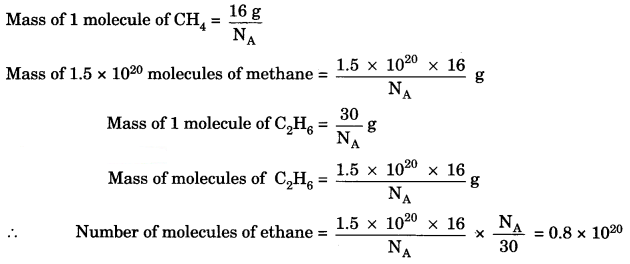Question 4.
Write the cations and anions present (if any) in the following compounds
(a) CH3COONa
(b) NaCl
(c) H2
(d) NH4NO3Question 5.
How many molecules of water are present in a drop of water which has a mass of 50 mg?
We know that:
1 mole of a compound = 6.023 × 1023 atoms
= Gram molecular mass
Gram molecular mass of H2O = 18 g
18 g = 6.023 × 1023 atomsQuestion 6.
Find the number of protons and neutrons in the nucleus of an atom of an element X which is represented as 207 X82.
The element is 207 X82.
Now, 82 = Atomic Number
207 = Mass Number.

(a) Atomic number = Number of protons
82 = Number of protons.

(b) Mass number = Number of protons + Number of neutrons
207 = 82 + Number of neutrons
207 – 82 = Number of neutrons
125 = Number of neutrons.

Question 7.
Calculate the molar mass of Na2SO4 and CaCO3.
Molar Mass of Na2SO4 =
2 × Mass of sodium + 1 × Mass of sulphur + 4 × Mass of oxygen
= 2 × 23 + 1 × 32 + 4 × 16
= 46 + 32 + 64
= 142 a.m.u.

Molar Mass of CaCO3 =
1 × Mass of calcium + 1 × Mass of carbon + 3 × Mass of oxygen
= 40 + 12 + 3 × 16
= 40 + 12 + 48
= 100 a.m.u.

Question 8.
What is wrong with the statement ‘1 mol of hydrogen’?
The statement is not correct. We must always write whether hydrogen is in atomic form or molecular form. The correct statement is 1 mole of hydrogen atoms or one mole of hydrogen molecules.

Question 9.
(a) Calculate the relative molecular mass of water (H2O). [NCERT Exemplar]
(b) Calculate the molecular mass of HNO3.
(a) Atomic mass of hydrogen = 1 u,
oxygen = 16 u
So the molecular mass of water, which contains two atoms of hydrogen and one atom of oxygen is
= 2 × 1 + 1 × 16 = 18u

(b) The molecular mass of HNO3 = the atomic mass of H + the atomic mass of N + 3 × the atomic mass of O
= 1 + 14 + 48 = 63 u

Question 10.
Identify the cations and anions in the following compounds:
(a) CH3COONa
(b) NH3
(c) NH4
(d) SrCl2
(a) Na+, C3H3COO
(b) It is a molecular compound
(c) NH4+, Cl
(d) Sr2+, 2Cl

Question 11.
Calculate the total number of electrons present in 1.6 g of methane.
Molecular mass of CH4 = 12+ 1 × 4 = 16 g
16 g = 1 mole
1.6 g = 0.1 mole
1 mole of CH4 has 6.02 × 1023 molecules.
0.1 mole has 6.02 × 1022 molecules.
1 molecule of methane contains (6 + 1 × 4) = 10 electrons
∴ 6.02 × 1022 molecule will contain = 10 × 6.02 × 1022 = 6.02 × 1023 electrons.

Question 12.
A 0.24 g sample of a compound of oxygen and boron was found by analysis to contain 0.096 g of boron and 0.144 g of oxygen. Calculate the percentage composition of the compound by weight.
% oi any element in a compound =% of boron = $$\frac { 0.096 }{ 0.24 }$$ × 100 = 40%
% of oxygen = $$\frac { 0.144 }{ 0.24 }$$ × 100 = 60%.

Question 13.
The percentage composition of sodium phosphate as determined by analysis is 42.1% sodium, 18.9% phosphorus and 39% oxygen. Find the empirical formula of the compound.(Work upto two decimal places).
(Atomic masses: Na = 23, P = 31, O = 16)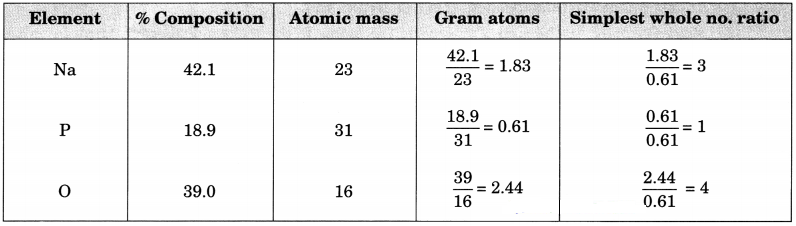Empirical formula = Na3PO4.

Question 14.
A sample of vitamin C is known to contain 2.58 × 1024 oxygen atoms. How many moles of oxygen atoms are present in the sample?
1 mole of oxygen atom = 6.023 × 1023 atoms
Number of moles of oxygen atoms = $$\frac{2.58 \times 10^{24}}{6.022 \times 10^{23}}$$ = 4.28 mol
4.28 moles of oxygen atoms.

Question 15.
The empirical formula of a compound is C2H4O. Its relative molecular mass is 88. Find the molecular formula.
Let the molecular formula be (C2H4O)n.
Molecular mass = n × empirical formula mass 88 = n × (2 × 12 + 4 × 1 + 16)
n = $$\frac { 88 }{ 44 }$$ = 2
The molecular formula is (C2H4O)2 = C4H8O2.

Question 16.
What is the mass in grams of one molecule of caffeine (C8H10N4O2)?
1 mole of caffeine molecules = Gram molecular mass of C8H10N4O2
= 8 × 12 + 10 × 1 + 4 × 14 + 2 × 16g
= 96 + 10 + 56 + 32 g = 194 g
Also, 1 mole of caffeine molecules = 6.022 × 1023 molecules
Thus, 6.022 × 1023 molecules of caffeine have mass = 194 g
1 molecule of caffeine will have mass = $$\frac{194}{6.022 \times 10^{23}}$$ g = 3.22 × 10-22

Question 17.
What is the difference between cation and anion?
Cation:

• It is positively charged, exam: Na+
• It is formed from metal atoms.
• On passing electric current, it moves towards cathode.
• It is smaller in size than its parent atom.

Anion:

• It is negatively charged, example : Cl
• It is formed from non-metal atoms.
• On passing electric current, it moves towards anode.
• It is larger in size than its parent atom.

Question 18.
Differentiate between:
(i) Atom and molecule.
(ii) Molecular mass and formula of unit mass.
(i) Atoms cannot exist independently but molecules can.

(ii) Molecular mass in the sum of masses of the atoms in the molecule whereas, formula unit mass is sum of atomic masses of the atoms in its empirical formula.

### Atoms and Molecules Class 9 Extra Questions Short Answer Type 2

Question 1.
Calcium chloride when dissolved in water dissociates into its ions according to the following equation.
CaCl2(aq) → Ca2+ (aq) + 2Cl(aq)
Calculate the number of ions obtained from CaCl2 when 222 g of it is dissolved in water. [NCERT Exemplar]
1 mole of calcium chloride = 111 g
222 g of CaCl2 is equivalent to 2 moles of CaCl2 Since 1 formula unit of CaCl2 gives 3 ions, therefore, 1 mol of CaCl2 will give 3 moles of ions.
2 moles of CaCl2 would give 3 × 2 = 6 moles of ions.
No. of ions = No. of moles of ions × Avogadro number
= 6 × 6.022 × 1023
= 36.132 × 1023
= 3.6 132 × 1024 ions.

Question 2.
Give the formulae of the compounds formed from the following sets of elements. [NCERT Exemplar]
(a) Calcium and fluorine
(b) Hydrogen and sulphur
(c) Nitrogen and hydrogen
(d) Carbon and chlorine
(e) Sodium and oxygen
(f) Carbon and oxygen
(a) CaF2
(b) H2S
(c) NH3
(d) CCl4
(e) Na2O
(f) CO, CO2

Question 3.
A compound was found to have the following percentage composition by mass Zn = 22.65%, S = 11.15%, H = 4.88%, O = 61.32%. The relative molecular mass is 287 g/mol. Find the molecular formula of the compound, assuming that all the hydrogen in the compound is present in water of crystallisation.
Zn : S : O : H = $$\frac { 22.65 }{ 65 }$$ : $$\frac { 11.15 }{ 32 }$$ : $$\frac { 61.32 }{ 16 }$$ : $$\frac { 4.88 }{ 1 }$$
= 0.3485 : 0.3484 : 3.833 : 4.88
To obtained an integral ratio, we divide by smallest number
= $$\frac { 0.3485 }{ 0.3484 }$$ : $$\frac { 0.3484 }{ 0.3484 }$$ : $$\frac { 3.833 }{ 0.3484 }$$ : $$\frac { 4.88 }{ 0.3484 }$$
= 1 : 1 : 11 : 14
∴ Empirical formula is Zn SO11H14.
Let molecules formula be (ZnSO11H14)n.
RMM for the molecules = n [65 + 32 + (11 × 16) + 14]
Formula = 287
287 n = 287
n = 1
∴ Molecular formula is ZnSO11H14.

Question 4.
What are the failures of Dalton Atomic theory?
Failures of Dalton Atomic Theory are:

1. Atom is not the smallest particle as it is made up of protons, neutrons and electrons.
2. Atom’s mass can be transformed to energy (E = mc2) and hence can be created and destroyed.
3. Atoms of one element have been changed into atoms of another element through artificial transmutation of elements.
4. Atoms of same element need not resemble each other in all respects as isotopes (different forms of same element) exist.
5. Atoms of different elements need not differ in all respects as isobars (same forms of different elements) exist.

Question 5.
The following questions are about one mole of sulphuric acid [H2SO4]?
(a) Find the number of gram atoms of hydrogen in it.
(b) How many atoms of hydrogen does it have?
(c) How many atoms (in grams) of hydrogen are present for every gram atom of oxygen in it?
(d) Calculate the number of atoms in H2SO4?
1 mole of H2SO4 = Gram Molecular mass = 6.023 × 1023 molecules
(a) In H2SO4 → 2 gram atoms of hydrogen are present

(b) One mole of H2SO4 contains = 6.023 × 1023 molecules
= 2 × 6.023 × 1023 atoms of H + 6.023 × 1023 atoms of S + 4 × 6.023 ×1023 atoms of O
∴ 2H = 2 × 6.023 × 1023
= 12.046 × 1023

(c) In H2SO4;
For every 2 hydrogen there are oxygen atoms
So for 1 hydrogen = $$\frac { 4 }{ 2 }$$ = oxygen are present
= 2 oxygen atoms are present o
For 1 oxygen = $$\frac { 2 }{ 4 }$$ hydrogen atoms are present
= 0.5 hydrogen atoms are present.

(d) 1 mole of H2SO4 = 6.023 × 1023 atoms

Question 6.
Write the formulae for the following and calculate the molecular mass for each one of them. [NCERT Exemplar]
(а) Caustic potash
(b) Baking powder
(c) Lime stone
(d) Caustic soda
(e) Ethanol
(f) Common salt.
(a) KOH
[39 + 16 + 1] = 56 g mol-1

(b) NaHCO3
23 + 1 + 12 + (3 × 16) = 84 mol-1

(c) CaCO3
40 + 12 + (3 × 16) = 100 g mol-1

(d) NaOH
23 + 16 + 1 = 40 g mol-1

(e) C2H6OH = C2H6O
2 × 12 + (6 × 1) + 16 = 46 g mol-1

(f) Nacl
23 + 35.5 = 58.5 g mol-1

Question 7.
Calculate the mass of the following: [NCERT Exemplar]
(i) 0.5 mole of N2 gas (mass from mole of molecule)
(ii) 0.5 mole of N atoms (mass from mole of atom).
(iii) 3.011 × 1023 number of N atoms (mass from number)
(iv) 6.022 × 1023 number of N2 molecules mass from number.
(i) mass = molar mass x number of moles
⇒ m = M × n = 28 x 0.5 = 14 g

(ii) mass = molar mass x number of moles
⇒ m = Mxn = 14x 0.5 = 7 g

(iii) The number of moles, n(iv) n = $$\frac{N}{N_{0}}$$
⇒ m = M x $$\frac{N}{N_{0}}$$ = 28 x $$\frac{6.022 \times 10^{2}}{6.022 \times 10^{2}}$$
= 28 x 1 = 28 g.

Question 8.
Calculate the number of particles in each of the following:
(i) 46 g of Na atoms (number from mass)
(ii) 8 g O2 molecules (number of molecules from mass)

(iii) 0.1 mole of carbon atoms (number from given moles)
(i) The numbers of atoms⇒ N = $$\frac { m }{ M }$$ x N0
⇒ N = $$\frac { 46 }{ 23 }$$ x 6.022 x 1023
⇒ N = 12.044 x 1023

(ii) The number of moleculesAtomic mass of oxygen = 16 u
molar mass of O2 molecules
= 16 x 2 = 32 g
⇒ N = $$\frac { 8 }{ 32 }$$ x 6.022 x 1023
⇒ N = 1.5055 x 1023
= 1.51 x 1023

(iii) The number of particles (atom) = numbers of moles of particles x Avogadro number
N = n x N0 = 0.1 x 6.022 x 1023.
= 6.022 x 1022.

Question 9.
A flask contains 4.4 g of CO2 gas. Calculate
(a) How many moles of CO2 gas does it contain?
(b) How many molecules of CO2 gas are present in the sample?
(c) How many atoms of oxygen are present in the given sample? (Atomic mass of C = 12 u, O = 16 u)
(a)(b) 1 mole of CO2 has molecules
= 6.022 x 1023
0. 1 mole of CO2 has molecules
= 6.022 x 1023 x (0.1) = 6.022 x 1022

(c) 1 mole of CO2 has oxygen atoms = 2 x N0
0. 1 mole of CO2 has oxygen atoms = 2 x N0 x 0.1
= 2 x 6.022 x 102 x 0.1 = 1.204 x 1024 atoms.

Question 10.
Calculate the number of moles for the following: [NCERT Exemplar]
(ii) 12.044 x 1023 number of He atoms (finding mole from number of particles).
No of moles = n
Given mass = m
Molar mass = M
Given number of particles = N
Avogadro number of particles = N0

(i) Atomic mass of He = 4 u
Molar mass of He = 4 g
Thus, the number of moles =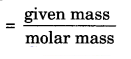= n = $$\frac { m }{ M }$$ = $$\frac { 52 }{ 4 }$$ = 13

(ii) We know,
1 mole = 6.022 x 1023
The number of moles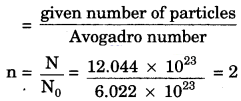Question 11.
Write the molecular formulae of all the compounds that can be formed by the combination of following ions:
Cu2+, Na+, Fe3+, Cb, SO42-, PO43-
(i) CuCl2, CuSO4, Cu3(PO4)2
(ii) NaCl, Na2SO4, Na3PO4
(iii) FeCl3, Fe2(SO4)3, FePO4

Question 12.
Give the names of any three elements whose names have been derived from Latin. Give their Latin names and symbols.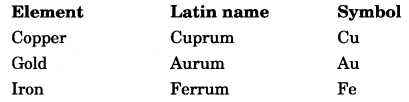Question 13.
What is the simplest formula of the compound which has the following percentage composition:
Carbon 80%, Hydrogen 20%
If the molecular mass is 30, calculate its molecular formula.
Calculation of empirical formula: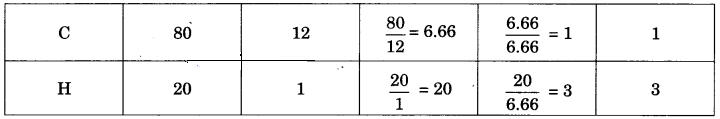∴ Empirical formula is CH3.
Calculation of molecular formula:
Empirical formula mass = 12 x 1 + 1 x 3 = 15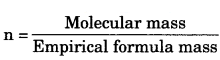$$\frac { 30 }{ 15 }$$ = 2
Molecular formula = Empirical formula x 2 = CH3 x 2 = C2H6.

Question 14.
On heating, potassium chlorate decomposes to potassium chloride and oxygen. In one experiment 30.0 g of potassium chlorate generates 14.9 g of potassium chloride and 9.6 g of oxygen. What mass of potassium chlorate remains undecomposed?
Potassium chlorate → Potassium chloride + Oxygen
Total mass of potassium chlorate before reaction = 30.0 g
After reaction, mass of potassium chloride = 14.9 g Mass of oxygen = 9.6 g
Let mass of undecomposed potassium chlorate = x g
Total mass after reaction = (x + 14.9 + 9.6) g
According to law of conservation of mass the total mass before and after the reaction remains constant.
∴ 30.0 = x + 14.9 + 9.6
x = 30 – 14.9 – 9.6 = 5.5
∴ Mass of undecomposed potassium chlorate
= 5.5 g

Question 15.
Naturally occurring Boron consists of two isotopes whose atomic mass are 10.01 and 11.01. The atomic mass of natural Boron is 10.81. Calculate the percentage of each isotope in natural Boron.
Suppose percentage of isotope with atomic mass 10.01 = x
Then percentage of isotope with atomic mass 11.01 = 100 – x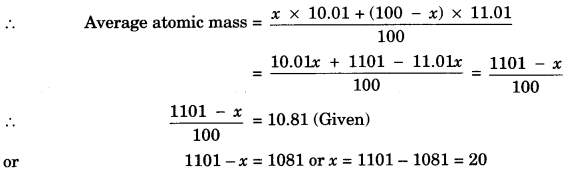∴ % of isotope with atomic mass 10.01 = 20%
∴ % of isotope with atomic mass 11.01 = 80%

Question 16.
Which amongst the following has more number of atoms, 11.5 g of sodium or 15 g of calcium?
How? (Given atomic mass of Na = 23, Ca = 40)
23 g of Na = 1 mole
11.5 g of Na = 11.5/23 mole
Now, 1 mole of Na contains = 6.022 x 1023 atoms
11.5/23 mole of Na contains = 6.022 x 1023 x 11.523 mole
= 3.011 x 1023 atoms
40 g of Ca = 1 mole
15 g of Ca = 15/40 moles
Now, 1 mole of Ca contains = 6.022 x 1023 atoms
15/40 mole of Ca contains = 6.022 x 1023 x 15/40 mole
= 2.258 x 1023 atoms
Na has more number of atoms.

Question 17.
(i) Name the body which approves the nomenclature of elements and compounds.
(ii) The symbol of sodium is written as Na and not as S. Give reason.
(iii) Name one element which forms diatomic and one which forms tetraatomic molecules.
(i) International Union of Pure and Applied Chemistry.

(ii) The symbol of Na (sodium) is derived from its Latin name Natrium.

(iii) (a) Element forming diatomic molecule: H2, O2, N2 (only one).
(b) Element forming tetratomic molecule: P4.

### Atoms and Molecules Class 9 Extra Questions Long Answer Type Questions

Question 1.
(a) Define empirical formula and molecular formula of a compound. How are molecular formula and empirical formula related to each other?
(b) A hydrocarbon contains 10.5 g of carbon per gram of hydrogen. Calculate the empirical formula.
(a) Empirical formula is the chemical formula which gives us the simplest whole number ratio between the atoms of various elements present in one molecule of a compound. Molecular formula gives us the actual number of atoms of various elements present in one molecule of a compound.
Molecular formula = n x Empirical formula
Where n is a simple whole number(b) In the hydrocarbon,
1 g of hydrogen combines with 10.5 g carbon
∴ % of H in hydrocarbon = $$\frac { 1 }{ 11.5 }$$ x 100 = 8.696%
% of C = 100 – 8.696 = 91.304%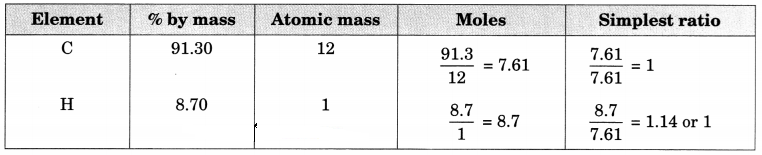Therefore, Empirical formula = CH

Question 2.
(a) What is meant by mole concept?
(b) Calculate the mass of:
(i) 1022 atoms of sulphur
(ii) 0.1 mole of carbon dioxide.
[Atomic mass S = 32, C = 12, O = 16 and Avogadro’s number = 6 x 1023]
(a) 1 mole of a compound has a mass equal to its relative molecular mass expressed in grams.
1 mole = 6.022 x 1023 number
= Relative mass in grams.

(b) (i) v 6.022 x 1023 atoms of sulphur weighs = 32 g
∴ 1022 atoms of sulphur weighs = $$\frac{32}{6.022 \times 10^{23}}$$ x 1022 = 0.531 g

(ii) Gram molar mass of CO2 = 12 + 2 x 16 = 44 g
∵ 1 mole of carbon dioxide weighs = 44 g
∴ 0.1 mole of carbon dioxide weighs = $$\frac { 44 g }{ 1 }$$ x 0.1 = 4.4 g.

Question 3.
(a) The ratio of hydrogen and oxygen in water is 1: 8 by mass, find out their ratio by number of atoms, in one molecule of water. (Atomic mass: H = 1 u, O – 16 u)
(b) Write the formulae of the following compounds:
(i) Ammonium sulphate
(ii) Magnesium chloride
(Given , ammonium = NH42+ , sulphate = SO42-, Magnesium = Mg2+, chloride = Cl)
(a)Ratio of number of atoms for water is H : O = 2 : 1

(b)
(i) Ammonium sulphate: (NH4)2SO4
(ii) Magnesium chloride : MgCl2

Question 4.
A compound of carbon and sulphur has a composition of 15.8% carbon and 84.2% sulphur.
(a) Find the empirical formula.
(b) The relative molecular mass of the compound is 76. Find the molecular formula.
(a)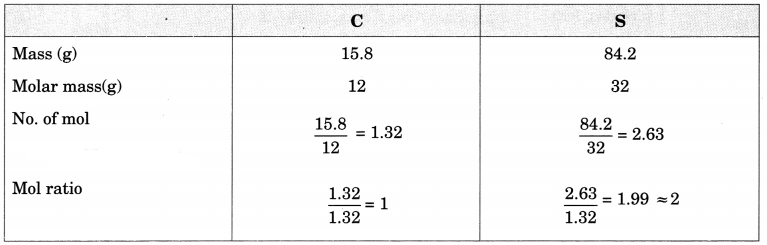The empirical formula is CS2.

(b) Let the molecular formula be (CS2)n.
Molecular mass = n x empirical formula mass
76 = n x (12 + 2 x 32)
n = $$\frac { 76 }{ 76 }$$ = 1
The molecule formula is (CS2)1 = CS2.

Question 5.
What do the following formulae stand for:
(a) (i) 20
(ii) O2
(iii) O3
(iv) H2O

(b) Give the chemical formulae of the following compounds?
(i) Potassium carbonate
(ii) Calcium chloride

(c) Calculate the formula unit mass of Al2(SO4)3.
(Given atomic mass of Al = 27u, S = 32 u, 0 = 16 u)
(a)
(i) Two atoms of oxygen 20
(ii) Diatomic oxygen → O2 molecule
(iii) Triatomic oxygen → O3 molecule
(iv) Two atoms of hydrogen and 1 atom of oxygen forming one molecule of water.

(b) K2CO3 : Potassium carbonate
CaC2 : Calcium chloride

(c) Al2(SO4)3
Al: 27 x 2 = 54 u
S : 32 x 3 = 96 u
O : 16 x 12 = 192 u
Formula unit mass = 342 u.

Question 6.
(a) Write the chemical symbols of two elements:
(i) Which are formed from the first letter of the elements name.
(ii) Whose name has been taken from the names of the elements in Latin.

(b) Define Avogadro’s constant and molar mass. How are they related to one mole of an atom, molecule, or ion?
(a)
(i) C, N, O, etc.
(ii) Na, Fe, K, etc.

6.22 x 1023 is defined as the Avogadro’s constant. It is number of atoms in exactly 12 g of carbon-12.

Molar mass : Mass of 1 mole of substance.Question 7.
(a) Calculate the mass of 0.5 mole of sulphuric acid. Atomic mass (H = 1 u, S = 32 u, O = 16 u).
(b) Find the number of atoms in 12 g of carbon.
(c) How many atoms are present in (i) H2S molecule (ii) PO3-4 ions.
(d) Write the names of elements present in (i) quicklime (ii) hydrogen bromide.
(a) 1 mole of H2SO4 = 98
∴ 1/2 mole of H2SO4 = 49 g

(b) 6.023 x 1023 atoms

(c) In H2S molecules, number of atoms = 3
In PO3-4 ion, number of atoms = 5.

(d) (i) Elements in quicklime (CaO)-calcium, oxygen.
(ii) Elements in hydrogen bromide (HBr)-hydrogen, bromine.

Question 8.
(a) Define polyatomic ions. Write an example.
(b) Calculate the formula unit mass of CaCO3.
(Atomic mass of C = 12 u, Ca = 40 u, O = 16 u)
(c) Calculate the molecular mass of the following:
(i) HNO3 (ii) CH3COOH
(Atomic mass of H = 1 u, N = 14 u, O = 16 u, C = 12 u)
(a) Cluster of atoms that acts as an ion are called polyatomic ions. example : NH4+, PO3-4, SO2-4, etc.

(b) CaCO3 = Mass of Ca + Mass of C + Mass of O
= 40 u + 12 u + 16 u x 3 = 100 u.

(c) (i) HNO3 = Mass of H + Mass of N + Mass of O
= 1 u + 14 u + 16 u x 3 = 63 u

(ii) CH3COOH = Mass of C + Mass of H + Mass of O
= 12 u x 2 + 1 u x 4 + 16 u x 2
= 24 u + 4 u + 32 u = 60 u

### Atoms and Molecules Class 9 Extra Questions HOTS

Question 1.
In photosynthesis, 6 molecules of carbon dioxide combine with an equal number of water molecules through a complex series of reactions to give a molecule of glucose having a molecular formula C6H12O6. How many grams of water would be required to produce 18 g of glucose? Compute the volume of water so consumed assuming the density of water to be 1 g cm-3. [NCERT Exemplar]1 mole of glucose needs 6 moles of water
180 g of glucose needs (6 x 18) g of water
1 g of glucose will need $$\frac { 108 }{ 180 }$$ g of water.
18 g of glucose would need $$\frac { 108 }{ 180 }$$ x 18 g of water = 10.8 g
Volume of water used = $$\frac { Mass }{ Density }$$ = $$\frac{10.8 \mathrm{g}}{1 \mathrm{g} \mathrm{cm}^{-3}}$$ = 10.8 cm3

Question 2.
Fill in the missing data in the Table 3.2. [NCERT Exemplar]
Table 3.2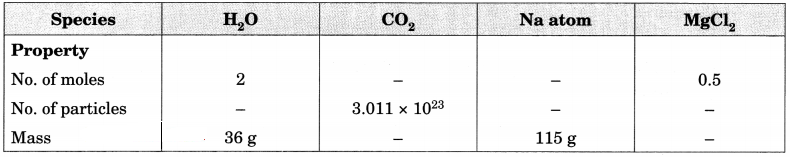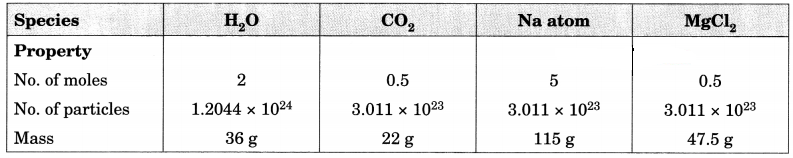Question 3.
Raunak took 5 moles of carbon atoms in a container and Krish also took 5 moles of sodium atoms in another container of same weight. [NCERT Exemplar]
(a) Whose container is heavier?
(b) Whose container has more number of atoms?
(a) Mass of sodium atoms carried by Krish = (5x 23) g = 115 g while mass of carbon atom carried by Raunak = (5 x 12) g = 60 g. Thus, Krish’s container is heavy.

(b) Both the bags have same number of atoms as they have same number of moles of atoms.

Question 4.
How many molecules are present in 1 ml. of water?
Molecular mass of H2O = 18 g
Mass of 1 mole of water = 18 g
18 g of water contain = 6.022 x 1023 molecules
Density of water = 1 g/ml, therefore 1 ml of water weighs 1 g.
1.0 g of water contain = (6.022 x 1023)/18 molecules
= 3.34 x 1022 molecules.

Question 5.
A flask P contains 0.5 mole of oxygen gas. Another flask Q contains 0.4 mole of ozone gas. Which of the two flasks contains greater number of oxygen atoms?
1 molecule of oxygen (O2) = 2 atoms of oxygen
1 molecule of ozone (O3) = 3 atoms of oxygen
In flask P:1 mole of oxygen gas = 6.022 x 1023 molecules
0.4 mole of oxygen gas = 6.022 x 1023 x 0.5 molecules
= 6.022 x 1023 x 0.5 x 2 atoms = 6.022 x 1023 atoms
In flask Q: 1 mole of ozone gas = 6.022 x 1023 molecules
0.4 mole of ozone gas = 6.022 x 1023 x 0.4 molecules
= 6.022 x 1023 x 0.4 x 3 atoms = 7.23 x 1023 atoms
∴ Flask Q has a greater number of oxygen atoms as compared to flask P.

Question 6.
Complete the following table: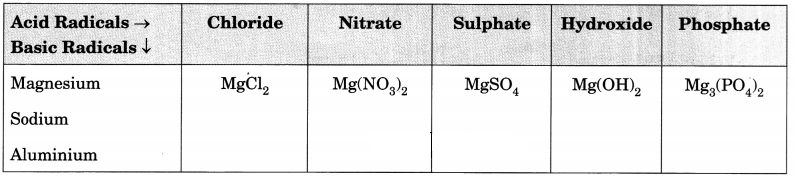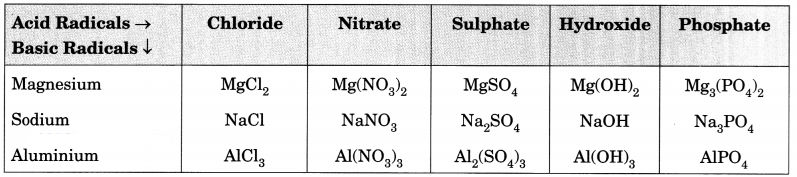Question 7.
What is the valency of underlined element in the following compounds?
(i) MnO2 (ii) Ca3N2 (iii) ZnSO4 (iv) CCl4
(i) + 4
(ii) + 2
(iii) + 2
(iv) + 4

Question 8.
A student puts his signature with graphite pencil. If the mass of carbon in the signature is 10-12 g. Calculate the number of carbon atoms in the signature.
Mass of carbon = 10-12 g
No. of moles of carbon = $$\frac{10^{-12}}{12}$$ moles
1 mole of C has = 6.022 x 1023
∵ 1 mole of C has atoms = 6.022 x 1023
∴ $$\frac{10^{-12}}{12}$$ mole of C will have = $$\frac{6.022 \times 10^{23}}{12}$$ x 1010

Question 9.
Rudra took 5 moles of carbon atoms in a container and Ayush also took 5 moles of sodium atoms in another container of some weight.
(a) Whose container is heavier?
(b) Whose container has more number of atoms?
(a) 1 mole of C atoms = 12 g
∴ 5 moles of C atoms = 5 x 12 g = 60 g
1 mole of Na atoms = 23 g
∴ 5 moles of Na atoms = 5 x 23 = 115 g
Thus, Ayush’s container is heavier.

(b) 1 mole of C atoms = 6.022 x 1023 atoms
1 mole of Na atoms = 6.022 x 1023 atoms
∴ 5 moles of each will contain the same number of atoms.

Question 10.
1022 atoms of an element ‘X’ are found to have a mass of 930 mg. Calculate the molar mass of the element ‘X’.
∴6.022 x 1023 atoms will have mass = $$\frac{0.930}{10^{22}}$$ x 6.022 x 1023 g = 56.0 g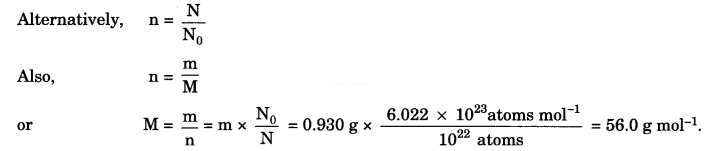No. of moles (n) in 1 g of gold = $$\frac { m }{ M }$$ = $$\frac { 1 }{ 197 }$$
No. of moles (n) in 1 g of silver = $$\frac { m }{ M }$$ = $$\frac { 1 }{ 108 }$$
Greater the number of moles, greater is the number of atoms present. As $$\frac { 1 }{ 197 }$$ > $$\frac { 1 }{ 108 }$$, silver will contain greater number of atoms.